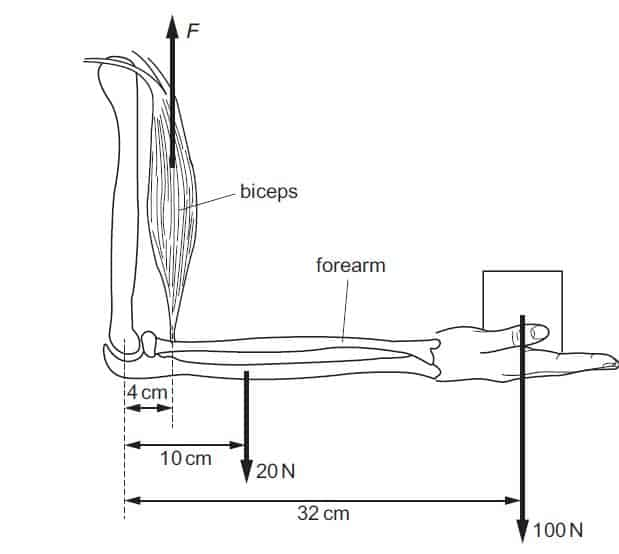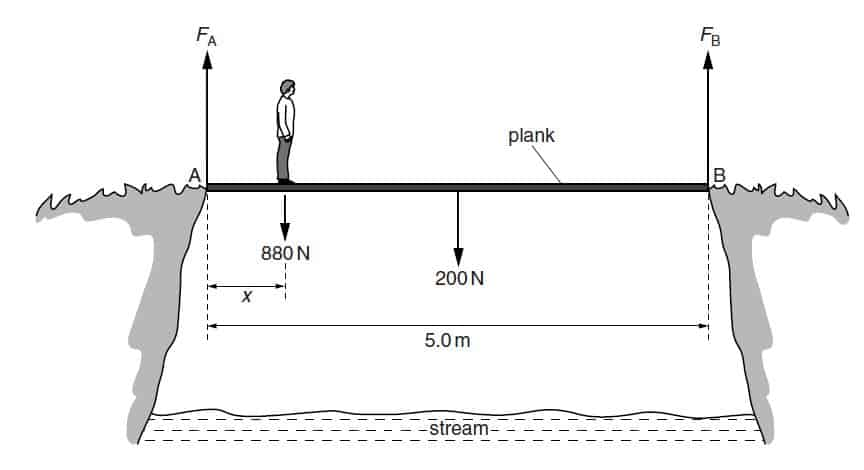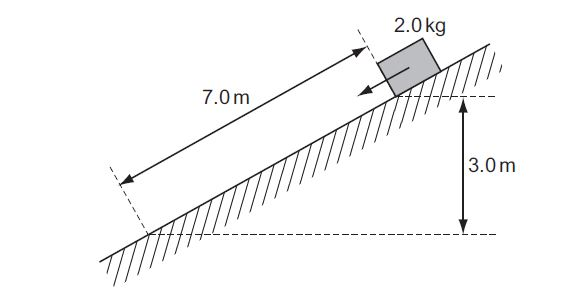Physic Tutorials

# How to solve questions on moment of a force

Question 1

A 90cm uniform lever has a load of 30N suspended at 15cm from one of its ends. If the fulcrum is at the centre of
gravity, the force that must be applied at its other end to keep it in horizontal equilibrium is{UME 2003 Type 9}
A. 15N                         B. 20N                          C. 30N                                   D. 60N.

Solutionsum of clockwise moment = sum of anti-clockwise moment

x * 45 = 30 * 30

45x = 900

x = 900 / 45

x = 20N

B is the correct option

Question 2

A 100kg box is pushed along a road with a force of 500N. If the box moves with a uniform velocity, the coefficient of
friction between the box and the road is{UME 2004 Type S}
A. 0.5                  B. 0.4                         C. 1.0                             D. 0.8

solution

F – fr = ma

since it moves with a uniform velocity acceleration = 0

F = fr

F = μR

μ is the coefficient of friction

F is the force applied

R is the normal reaction which is equal to the weight = mg = 100*10 = 1000N

μ = F/R  = 500 / 1000 = 0.5

A is the correct option

Question 3

A man holds a 100 N load stationary in his hand. The combined weight of the forearm and hand is
20 N. The forearm is held horizontal, as shown{cambridge may/june 2014 p11 ques 12}What is the vertical force F needed in the biceps?
A 750 N                  B 800 N                      C 850 N                                    D 900 N

Solution

moment of a force = force x perpendicular distance

Sum of clockwise moment = sum of anti-clockwise moment

the 100N load will make a clockwise direction

the 20N combined weight of the hand and forearm will make a clockwise direction

the force F in the biceps will make anti-clockwise direction

F*4 = 20*10 + 100*32

4F = 200 +3200

4F = 3400

f = 3400/4

F = 850N

C is the correct option

Question 4

A uniform plank AB of length 5.0 m and weight 200 N is placed across a stream, as shown belowA man of weight 880 N stands a distance x from end A. The ground exerts a vertical force FA on the plank at end A and a vertical force FB on the plank at end B.
As the man moves along the plank, the plank is always in equilibrium.{cambridge may/jun 2014 p21 q3}

The man stands a distance x = 0.50 m from end A. Use the principle of moments to
calculate the magnitude of FB.

Solution

sum of clockwise moment = sum of anti-clockwise moment

since it is a uniform plank, the weight of the plank will be at the mid-point of the plank

FB will make a anticlockwise direction

the man will make an clockwise direction

the weight of the plank will make clockwise direction

FB*5 = 200*2.5 +880*0.5

5FB = 500 +440

5FB = 940

FB = 940 / 5

FB = 188N

Question 5

A block of mass 2.0 kg is released from rest on a slope. It travels 7.0 m down the slope and falls a vertical distance of 3.0 m. The block experiences a frictional force parallel to the slope of 5.0 N.{cambridge may/june 2011 p11 que15}What is the speed of the block after falling this distance?
A 4.9 m s–1                   B 6.6 m s–1                       C 8.6 m s–1                               D 10.1 m s–1

Solution

mgsinθ – fr = ma

sinθ = opposite / hypothenus = 3 /7

g = 9.81 ms-2

2*9.81*3/7   –  5 = 2*a

8.41 – 5 = 2a

3.41 = 2a

a = 3.41 /2

a = 1.7 ms-2

using

v2 = u2 + 2as

initially it is at rest so

u = 0ms-1

distance covered is 7m

v2 = 2*1.7*7

v2 = 23.86

find the square root of both sides

v = 4.9ms-1

A is the correct option

Pls share to your friends and love ones if you find this interesting

### Bolarinwa Olajire

A lecturer, Educationist, PhD student at FUNAAB, and a Blogger.